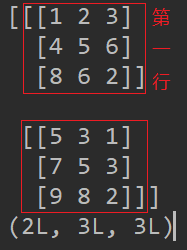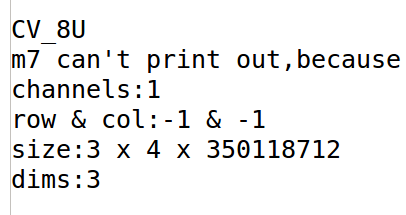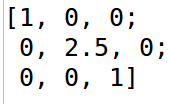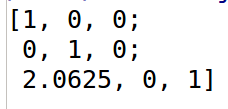# OpenCV中的Mat数据类型学习笔记

## Aug 24,2019   15288 words   55 min

#### 1.Mat创建

##### (1)使用构造函数或`create()`成员函数

Mat构造函数很多，经典的构造函数的格式如下：`Mat(nrows,ncols,type[,fillValue])`，中括号里代表可选参数，表示填入的初始值，如果不写默认为0。`nrows``ncols`一般为`int`型的整数，但`ncols`也可以为数组，这样表示建立一个多维`Mat`,此时传入的`nrows`表示维度。前两个参数还可以用`Size(ncol,nrow)`代替(注意`Size`里行列的顺序和函数参数的顺序是反的)，这就是另一种构造函数了。`create()`成员函数格式如下：`Mat(nrows,ncols,type)`，它只能接收3个参数，与构造函数的区别就是无法赋初值。使用示例如下：

``````#include <iostream>
#include <opencv2/opencv.hpp>

using namespace cv;
using namespace std;

int main() {
Mat m1(4, 3, CV_8UC1);
cout << m1 << endl << endl;

Mat m2(3, 5, CV_8UC1, 200);
cout << m2 << endl << endl;

Mat m3(2, 4, CV_8UC1, -10);
cout << m3 << endl << endl;

Mat m4;
m4.create(2, 3, CV_8UC1);
cout << m4 << endl;
cout << "CV_8UC1" << endl;
cout << "channels:" << m4.channels() << endl;
cout << "row & col:" << m4.rows << " & " << m4.cols << endl;
cout << "size:" << m4.size << endl;
cout << "dims:" << m4.dims << endl << endl;

Mat m5(3, 2, CV_8UC3, Scalar(20, 10, 30));
cout << m5 << endl;
cout << "CV_8UC3" << endl;
cout << "channels:" << m5.channels() << endl;
cout << "row & col:" << m5.rows << " & " << m5.cols << endl;
cout << "size:" << m5.size << endl;
cout << "dims:" << m5.dims << endl << endl;

Mat m6(3, 2, CV_8UC(5));
cout << m6 << endl;
cout << "CV_8UC5" << endl;
cout << "channels:" << m6.channels() << endl;
cout << "row & col:" << m6.rows << " & " << m6.cols << endl;
cout << "size:" << m6.size << endl;
cout << "dims:" << m6.dims << endl << endl;

int sz[] = {3, 4, 2};
Mat m7(3, sz, CV_8U, Scalar::all(0));
cout << "CV_8U" << endl;
cout << "m7 can't print out,because m7.dims = 3 and it requires m.dims <= 2" << endl;
cout << "channels:" << m7.channels() << endl;
cout << "row & col:" << m7.rows << " & " << m7.cols << endl;
cout << "size:" << m7.size << endl;
cout << "dims:" << m7.dims << endl << endl;
return 0;
}
``````

`m1`按照常规构造函数的方式新建且没有设初值，所以建好后元素全为0。

`m2`也是按常规构造函数新建，不同的是设置了初值200，所以输出结果矩阵元素全为200。

`m3`采用构造函数方式构建，不同的是初值设置。由于设置的数据类型是`8UC1`(8bit无符号整形，1个通道)，因此取值范围是0-255，而-10越界了，所以结果取最小值0。同理若超过255，所有元素取255，这点需要注意。

`m4`按照成员函数方式构建，其不能给矩阵设初值。而且有个非常值得注意的问题是如果在它之前新建了其它的`Mat`，那么它的初始值是随机的，而并非全为0。只有当它是第一个被新建的时候，初始值才全为0。在本段代码中，只有把新建`m4`之前的代码注释掉后，才全为0。尚不清楚为什么会出现这个情况。在官方文档中有这样一段解释，可能有关：create() allocates only a new array when the shape or type of the current array are different from the specified ones.

`m5`按照构造函数方式构建，不同的是数据类型为`8UC3`(8bit无符号整形，3个通道)，因此赋初值的时候需要注意，不能再直接写一个数字。如果写了，默认就是对第一个通道赋值。在有多个通道的时候，正确的赋值方法是用`Scalar()`函数传入数值，传入的数值分别对应各通道的初值。严格来说在只有一个通道的时候最严谨的写法是`Scalar(200)`，但为了方便也可以不这样写。需要特别注意的是`Scalar`的构造函数最多只支持4个数(通道)，如果多于4个数(通道)会报错提示找不到对应的构造函数，即使用`Scalar::all()`也不行。对于多于4个通道的`Mat`可以在新建好后，利用循环迭代赋值。另外需要注意的是控制台中对于多通道`Mat`的输出方式。由于不同通道设置了不同的初值，所以可以比较容易看出它的输出规律。简单来说就是行数与我们设置的相同，每行用封号隔开；在每行内，逐元素按照通道依次输出。例如本代码中输出的结果：第一行第一个元素的通道1的值、通道2的值、通道3的值；第一行第二个元素的通道1的值、通道2的值、通道3的值；第二行第一个元素的通道1的值、通道2的值、通道3的值…以此类推。 这种储存方法与Numpy对于多层数据的表达类似。Numpy对于有多层的数据存储方式是把每一个位置的所有通道的值都存成一个向量，然后再是下一个元素，注意理解。例如如下所示矩阵：该矩阵共有2行、3列、3个通道，其中[1 2 3]表示第一行第一列的位置在3个通道中的值，同理[4 5 6]表示第一行第二列位置在3个通道中的值，[8 6 2]表示第一行第三列的位置在三个通道中的值，[5 3 1]表示第二行第一列位置对应三通道的值。 可以理解为先构造好了一个2行3列的矩阵，然后获取每个位置在不同波段中对应的值组成一个向量，最后放在这个位置上，用向量替换单一的数字。

`m6`也是通过构造函数新建了一个数据类型为8U的`Mat`。这里由于没有赋初值，也出现了随机数问题，解决方法与之前一样。但与之前不同用的是 `CV8UC()`函数，其括号里的数字表示通道数，可以为任意数字，如20、30等，如`CV8UC(3)`等价于`CV8UC3`。这对于处理高光谱影像非常有用。注意由于代码中的`Mat`其通道数超过了4，所以无法使用`Scalar`赋初值，可手动循环赋值。

`m7`依旧采用了构造函数方式创建，但创建的`Mat`比较特别，是一个五维的矩阵块(?)，想象不出来了。第一个参数表示创建的矩阵维度为5维，第二个参数为整型数组表示每一维的大小，第三个参数表示数据类型，最后调用了`Scalar`赋初值。注意`sz[]`数组一定要和第一个参数设置的维度对应，不能多也不能少。经过测试，多了不会报错，最终效果是只取数组中前几个维数个数元素，后面不管了。但如果少了，问题比较大，比如数组里为3、4，而设置的还是3维，这样就等于第三维没设置，程序运行的时候就会设置一个非常大的数，如下(为了说明这个问题，电脑差点跑崩溃)这里再简单解释下OpenCV中对于`Mat`维度的规定，其规定`Mat`的维度永远是>=2的，即使是一维的数组，其也认为是nx1的矩阵。所以在这里如果你第一个参数传入的是1,最终得到的矩阵的`dims`属性还是会为2。

##### (2)使用拷贝构造函数或赋值运算符

``````#include <iostream>
#include <opencv2/opencv.hpp>

using namespace cv;
using namespace std;

int main() {
Mat m1(Size(3, 2), CV_16S, Scalar(-3));
Mat m2(m1);

Mat m3 = m1;
Mat m5 = m1.clone();

m3.at<short>(1, 1) = 2;
m5.at<short>(0, 1) = 9;
cout << "m1:" << endl << m1 << endl << endl;
cout << "m2:" << endl << m2 << endl << endl;
cout << "m3:" << endl << m3 << endl << endl;
cout << "m5:" << endl << m5 << endl;
return 0;
}
``````

##### (3)块操作赋值

``````#include <iostream>
#include <opencv2/opencv.hpp>

using namespace cv;
using namespace std;

int main() {
Mat m1 = Mat::eye(3, 5, CV_16S);
cout << m1 << endl << endl;

Mat m2(m1, Rect(1, 0, 3, 2));
cout << m2 << endl << endl;

m2.at<short>(0, 1) = 5;
cout << m2 << endl << endl;
cout << m1 << endl << endl;

Mat m3 = m1(Range::all(), Range(1, 3)).clone();
cout << m3 << endl << endl;
m3.at<short>(1, 1) = 9;
cout << m3 << endl << endl;
cout << m1 << endl;
return 0;
}
``````

##### (4)通过二维数组转换

``````#include <iostream>
#include <opencv2/opencv.hpp>

using namespace cv;
using namespace std;

int main() {
double m = {1, 2, 3, 4, 5, 6, 7, 8, 9};
Mat mat = Mat(3, 3, CV_64F, m);
cout << mat << endl;
return 0;
}
``````
##### (5)通过CvMat转换

``````#include <iostream>
#include <opencv2/opencv.hpp>

using namespace cv;
using namespace std;

int main() {
Mat img = cvarrToMat(iplimg);
return 0;
}
``````
##### (6)类Eigen赋值法

``````template<typename _Tp> inline
Mat_<_Tp>::Mat_(int _rows, int _cols)
: Mat(_rows, _cols, traits::Type<_Tp>::value)
{
}
``````

``````#include <iostream>
#include <opencv2/opencv.hpp>

using namespace cv;
using namespace std;

int main() {
Mat m = (Mat_<double>(3, 3) << 1, 0, 0, 0, 1, 0, 0, 0, 1);
cout << m << endl;
cout << m.at<double>(0, 1) << endl;
return 0;
}
``````

#### 2.Mat元素操作

``````#include <iostream>
#include <opencv2/opencv.hpp>

using namespace cv;
using namespace std;

int main() {
Mat m = Mat::eye(3, 3, CV_64F);
m.at<double>(1, 1) = 2.5;
cout << m << endl;
return 0;
}
``````

``````template<> class DataType<bool>
{
public:
typedef bool        value_type;
typedef int         work_type;
typedef value_type  channel_type;
typedef value_type  vec_type;
enum { generic_type = 0,
depth        = CV_8U,
channels     = 1,
fmt          = (int)'u',
type         = CV_MAKETYPE(depth, channels)
};
};

template<> class DataType<uchar>
{
public:
typedef uchar       value_type;
typedef int         work_type;
typedef value_type  channel_type;
typedef value_type  vec_type;
enum { generic_type = 0,
depth        = CV_8U,
channels     = 1,
fmt          = (int)'u',
type         = CV_MAKETYPE(depth, channels)
};
};

template<> class DataType<schar>
{
public:
typedef schar       value_type;
typedef int         work_type;
typedef value_type  channel_type;
typedef value_type  vec_type;
enum { generic_type = 0,
depth        = CV_8S,
channels     = 1,
fmt          = (int)'c',
type         = CV_MAKETYPE(depth, channels)
};
};

template<> class DataType<char>
{
public:
typedef schar       value_type;
typedef int         work_type;
typedef value_type  channel_type;
typedef value_type  vec_type;
enum { generic_type = 0,
depth        = CV_8S,
channels     = 1,
fmt          = (int)'c',
type         = CV_MAKETYPE(depth, channels)
};
};

template<> class DataType<ushort>
{
public:
typedef ushort      value_type;
typedef int         work_type;
typedef value_type  channel_type;
typedef value_type  vec_type;
enum { generic_type = 0,
depth        = CV_16U,
channels     = 1,
fmt          = (int)'w',
type         = CV_MAKETYPE(depth, channels)
};
};

template<> class DataType<short>
{
public:
typedef short       value_type;
typedef int         work_type;
typedef value_type  channel_type;
typedef value_type  vec_type;
enum { generic_type = 0,
depth        = CV_16S,
channels     = 1,
fmt          = (int)'s',
type         = CV_MAKETYPE(depth, channels)
};
};

template<> class DataType<int>
{
public:
typedef int         value_type;
typedef value_type  work_type;
typedef value_type  channel_type;
typedef value_type  vec_type;
enum { generic_type = 0,
depth        = CV_32S,
channels     = 1,
fmt          = (int)'i',
type         = CV_MAKETYPE(depth, channels)
};
};

template<> class DataType<float>
{
public:
typedef float       value_type;
typedef value_type  work_type;
typedef value_type  channel_type;
typedef value_type  vec_type;
enum { generic_type = 0,
depth        = CV_32F,
channels     = 1,
fmt          = (int)'f',
type         = CV_MAKETYPE(depth, channels)
};
};

template<> class DataType<double>
{
public:
typedef double      value_type;
typedef value_type  work_type;
typedef value_type  channel_type;
typedef value_type  vec_type;
enum { generic_type = 0,
depth        = CV_64F,
channels     = 1,
fmt          = (int)'d',
type         = CV_MAKETYPE(depth, channels)
};
};
``````

``````#define CV_8U   0
#define CV_8S   1
#define CV_16U  2
#define CV_16S  3
#define CV_32S  4
#define CV_32F  5
#define CV_64F  6
#define CV_USRTYPE1 7
``````

``````typedef Vec<uchar, 3> Vec3b;
typedef Vec<short, 3> Vec3s;
typedef Vec<ushort, 3> Vec3w;
typedef Vec<int, 3> Vec3i;
typedef Vec<float, 3> Vec3f;
typedef Vec<double, 3> Vec3d;

``````

bool CV_8U 0 -
uchar CV_8U 0 VecXb
schar CV_8S 1 -
char CV_8S 1 -
ushort CV_16U 2 VecXw
short CV_16S 3 VecXs
int CV_32S 4 VecXi
float CV_32F 5 VecXf
double CV_64F 6 VecXd

``````#include <iostream>
#include <opencv2/opencv.hpp>

using namespace cv;
using namespace std;

int main() {
Mat m = Mat::eye(3, 3, CV_32F);
m.at<double>(1, 1) = 2.5;
cout << m << endl;
return 0;
}
``````

`Mat``32F`也就是`float`类型，所以应该为`at<float>(1,1)`，正确结果如下：但这里写成了`double`，输出结果如下：可以看到由于数据类型不同导致了完全错误的结果(已经不是`float``double`精度不同的问题了，数值、位置全不一样)。这样的原因是OpenCV是根据元素所在位置(行列号)以及数据类型所占内存空间来计算每个元素所对应的内存地址的。而现在数据类型所占内存空间错了就会导致算出来的元素内存地址有问题，从而出现这种情况。

[2021-02-25更新]

``````Mat mat1(10, 10, CV_8UC1, 30);
Mat mat2;
// 直接调用convertTo函数进行类型转换
mat1.convertTo(mat2, CV_32FC1);
// 调用type函数查看数据类型
cout << mat1.type() << endl;
cout << mat2.type() << endl;
``````

[更新结束]

#### 3.Mat的遍历

##### (1)`ptr()`函数

``````#include <iostream>
#include <opencv2/opencv.hpp>

using namespace cv;
using namespace std;

int main() {
Mat M = Mat::eye(40, 40, CV_64F);
double sum = 0;
for (int i = 0; i < M.rows; i++) {
const double *Mi = M.ptr<double>(i);
for (int j = 0; j < M.cols; j++)
sum += std::max(Mi[j], 0.);
}
cout << sum << endl;
return 0;
}
``````

``````#include <iostream>
#include <opencv2/opencv.hpp>

using namespace cv;
using namespace std;

int main() {
Mat m(100, 100, CV_64FC(32));
RNG rng;
int row = m.rows;
int col = m.cols * m.channels();
for (int i = 0; i < row; ++i) {
double *data = m.ptr<double>(i);
for (int j = 0; j < col; ++j) {
data[j] = rng.uniform(0, 2048) / 2.0;
}
}
return 0;
}
``````

[2021-02-25更新]

``````Mat a(32, 32, CV_32FC3);
// 实例化一个随机数发生器
RNG rng;
// 使用fill方法
rng.fill(a, RNG::UNIFORM, 0.f, 1.f);
// 输出结果
cout << a << endl;
``````

OpenCV的API中，本身并没有一步到位很方便的随机矩阵生成接口，所以需要Mat搭配RNG使用。更详细的介绍可以参考这个网页

[更新结束]

##### (2)`at()`函数

``````#include <iostream>
#include <opencv2/opencv.hpp>

using namespace cv;
using namespace std;

int main() {
Mat img2 = img.clone();
for (int i = 0; i < img2.rows; ++i) {
for (int j = 0; j < img2.cols; ++j) {
if (img2.at<Vec3b>(i, j) > 128) {
img2.at<Vec3b>(i, j) = Vec3b(255, img2.at<Vec3b>(i, j), img2.at<Vec3b>(i, j));
} else {
}
}
}
imshow("img", img);
imshow("img2", img2);
waitKey(0);
return 0;
}
``````

#### 3.一些常用属性或函数

• `.channels()`：返回`Mat`的通道个数

• `.row()`：返回`Mat`的某一行数据(可修改)

• `.col()`：返回`Mat`的某一列数据(可修改)

• `.rows`：返回`Mat`的行数，仅对二维`Mat`有效，高维返回-1

• `.cols`：返回`Mat`的列数，仅对二维`Mat`有效，高维返回-1

• `.at()`：返回`Mat`某行某列的元素(可修改)

• `.ptr()`：返回指向`Mat`的行指针

• `.size`：返回`Mat`的尺寸大小(长x宽x高…)

• `.dims`：返回`Mat`中数据的维度，OpenCV中维度永远大于等于2

• `.clone()`：返回`Mat`的深拷贝

• `.copyTo()`：返回`Mat`的深拷贝

• `.eye()`：生成单位阵

• `.zeros()`：生成元素全为0的矩阵

• `.ones()`：生成元素全为1的矩阵

• `.type()`：返回`Mat`的元素类型索引

• `.inv()``Mat`求逆

• `.mul()``Mat`矩阵乘法

• `.data()`：返回指向矩阵数据单元的指针

• `.total()`：返回矩阵中的元素总数

• `cv::hconcat()`：水平拼接两个矩阵

• `cv::vconcat()`：数值拼接两个矩阵

#### 4.参考资料

• https://docs.opencv.org/3.1.0/d3/d63/classcv_1_1Mat.html
• https://blog.csdn.net/mao_hui_fei/article/details/78412301